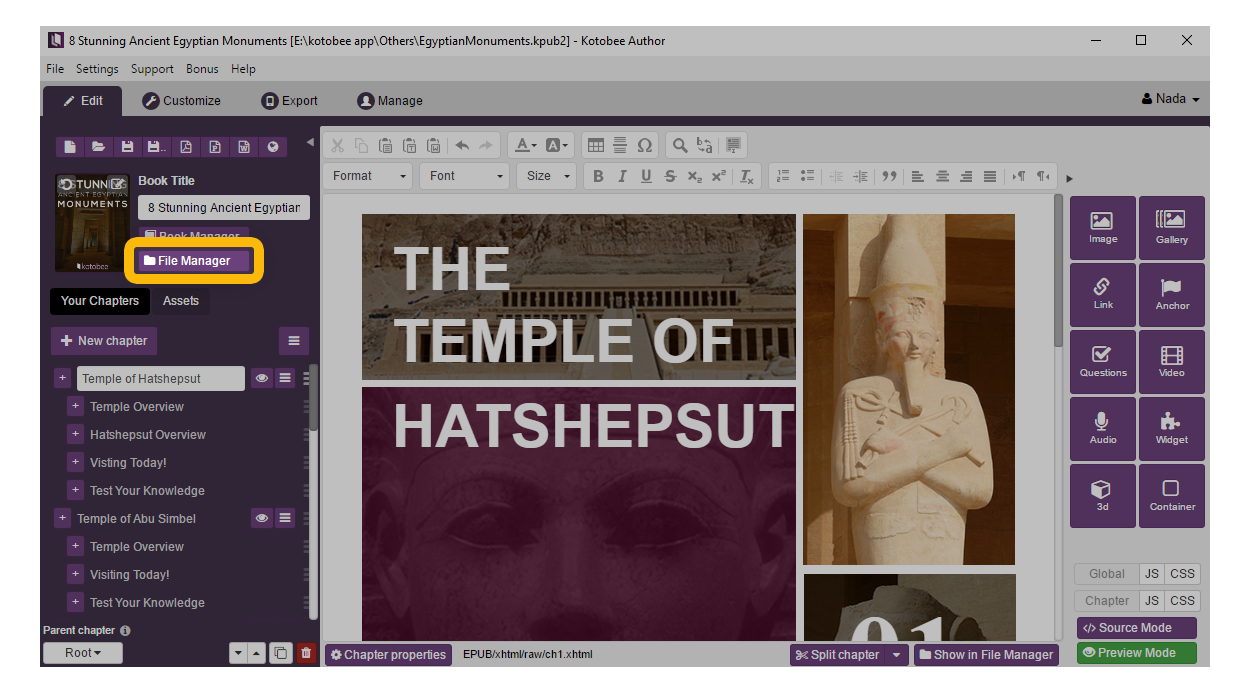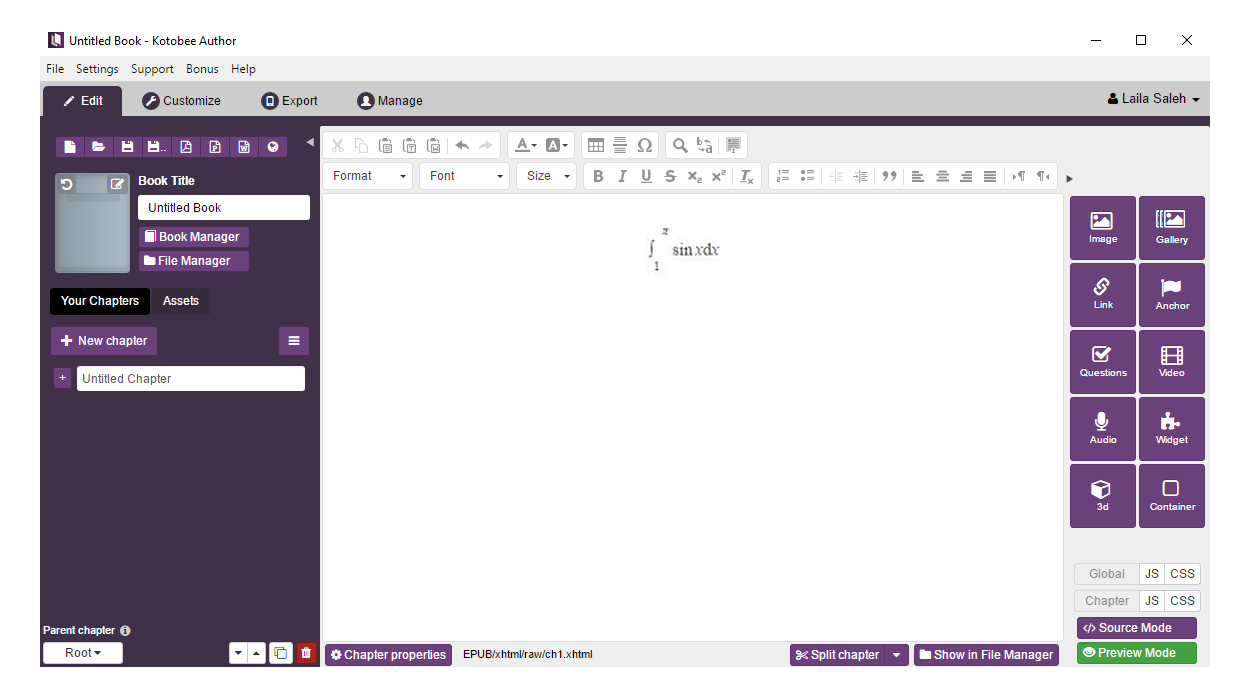Learn how to write mathematical expressions as text in your ebook. We will be using the KaTeX JavaScript library.

To write mathematical expressions as text within your ebook we will need to add an external JavaScript library. There are many libraries out there that help you do just that, but the specific library we will be using for this tutorial is KaTeX, which is a JavaScript library for displaying math connotations on web browsers. It is based on LaTeX, which means you’ll benefit from it loading very fast, and you’ll be writing mathematical expressions and formulas with the LaTeX language, as shown in the image below.# Import the necessary KaTeX files

2. Open Kotobee Author's File Manager.3. Import from the downloaded zip folder just three files to their appropriate folders:
• katex.min.js  to  EPUB > JS
• contrib > auto-render.min.js  to  EPUB > JS
• katex.min.css  to  EPUB > CSS# Using KaTeX In Your Ebook

1. Go back to the Edit Screen.
2. Go into Source mode to get the HTML of the ebook.
3. Copy-paste the following code inside the <head> tag to include the libraries we just imported.
<script type="text/javascript" src="../../js/katex.min.js"></script><script type="text/javascript" src="../../js/auto-render.min.js"></script><link type="text/css" rel="stylesheet" href="../../css/katex.min.css" /><script>    function ready(){    renderMathInElement(document.getElementById("kotobee") || document.body, {delimiters: [ // mind the order of delimiters(!?)    {left: "$", right: "$", display: true},    {left: "$", right: "$", display: false},    {left: "\$", right: "\$", display: true},    {left: "\$$", right: "\$$", display: false},    ]});    }    </script>

4. Change the body tag as follows, to have the equation rendered and not simply show the LaTeX connotations. We will execute the rendering function inside a function called ready() and have the body of the HTML run it first by adding onload=”ready()” to its tag.
<body onload="ready()">

5.  Add class="parsed" to the paragraph tag, to parse the text inside the editor.  Be certain to include the appropriate tags around your equation $Your equation$, so the complete tags should look like this example of the integral of sinx with respect to x over the domain of 1 to π:
<p class="parsed">$\int_1^\pi \sin x \mathrm{d} x$</p>If you’re not certain how to write your equation in LaTeX format, there are online editors that will translate it for you, then you can copy paste it, into your source in Kotobee.